# Assignments

In order to solve quadratic inequalities, it is necessary that you know how to solve quadratic equations, see also Quadratic equations (abc-formula) and Quadratic equations (factorizing). An important quantity is the discriminant. Following a series of assignments in which these aspects are important.

1. Solve: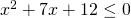Solution

2. Solve: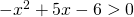Solution

3. Solve: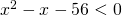Solution

4. Solve:Solution

5. Solve: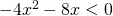Solution

6. Solve: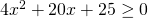Solution

7. Solve: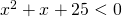Solution

8. For which value(s) ofdoes the following inequality hold: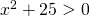Solution

9. For which value(s) ofdo the parabola: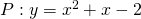and the line: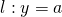have no points in common?

Solution

10. For which value(s) ofdo the parabola: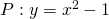and the line: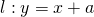have no points in common?

Solution

0